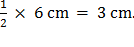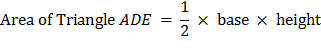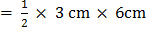### Sample Problem

ABCE is a rectangle. AE = AB = 6 cm and CD = CE. Find the area of the shaded part of ABCE.The area of the shaded part of ABCE is cm2.

#### Solution

Solution:

First, find the area of ABCE.

AE = AB = 6cm

Area of rectangle ABCE (which is actually a square) = length × width

= 6 cm × 6 cm

= 36 cm2.

Next, find the area of ADE.

ED = CD =CE == 9 cm2.

The area of ADE is 9 cm2.

Then, to find the area of the shaded part, we take the area of ABCE and subtract the area of ADE.

Area of shaded part = Area of ABCE – Area of ADE

= 36 cm2 – 9cm2

= 27 cm2.

The area of shaded part of ABCE is 27 cm2.